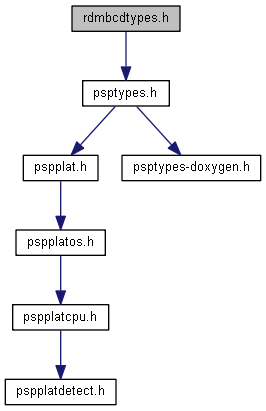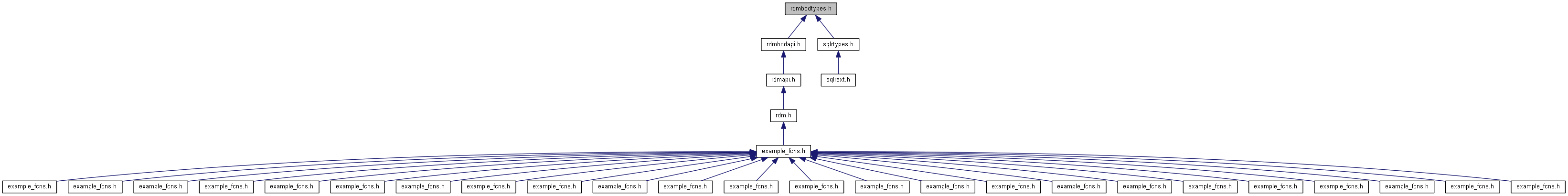rdmbcdtypes.h File Reference

Header for Binary-coded decimal (BCD) types. More...

`#include "psptypes.h"`
Include dependency graph for rdmbcdtypes.h:This graph shows which files directly or indirectly include this file:## Data Structures

struct RDM_BCD_T
The RDM Binary Coded Decimal (BCD) data structure. More...

## Macros

#define RDM_BCDLEN(prec) ((uint16_t) (offsetof (RDM_BCD_T, data) + (prec) + 1))
The length of a RDM BCD in bytes given by its precission. More...
#define RDM_BCD_MAXPRECISION 32
The max presission for a RDM BCD. More...
#define RDM_BCD_MAXSCALE 32

## Enumerations

enum RDM_BCD_ROUNDING_MODE {
RDM_BCD_ROUND_NEAREST_TIES_TO_EVEN, RDM_BCD_ROUND_NEAREST_TIES_AWAY_FROM_ZERO, RDM_BCD_ROUND_UP, RDM_BCD_ROUND_DOWN,
RDM_BCD_ROUND_TOWARDS_ZERO
}
Rounding modes for RDM BCD. More...

## Detailed Description

Header for Binary-coded decimal (BCD) types.

## RDM_BCD_MAXPRECISION

 #define RDM_BCD_MAXPRECISION 32

The max presission for a RDM BCD.

## RDM_BCD_MAXSCALE

 #define RDM_BCD_MAXSCALE 32

## RDM_BCDLEN

 #define RDM_BCDLEN ( prec ) ((uint16_t) (offsetof (RDM_BCD_T, data) + (prec) + 1))

The length of a RDM BCD in bytes given by its precission.

## RDM_BCD_ROUNDING_MODE

Rounding modes for RDM BCD.

Enumerator
RDM_BCD_ROUND_NEAREST_TIES_TO_EVEN

This is the default (because it is the recommended default for decimal numbers in IEEE 754). When rounding is necessary, the digits that are to be discarded are investigated and rounding takes place towards the nearer value. If these digits put the value exactly in the middle (i.e., the first digit to be truncated is 5 and all other truncated digits are 0) then the digit before the truncated digits is investigated and if it is even the data is rounded towards it - otherwise it is rounded away from it. The idea is to minimize rounding bias by rounding one way half of the time and rounding the other way the other half.

RDM_BCD_ROUND_NEAREST_TIES_AWAY_FROM_ZERO

This is what most of us are familiar with for rounding.Similar to the previous one, but 'ties' (when the truncated digits are 50000...) are rounded away from zero.

RDM_BCD_ROUND_UP

All values are rounded up; meaning positive values are rounded away from zero and negative values are rounded towards zero.

RDM_BCD_ROUND_DOWN

All values are rounded down; meaning positive values are rounded towards zero and negative values are rounded away from zero.

RDM_BCD_ROUND_TOWARDS_ZERO

All values are rounded towards zero.

@ RDM_BCD_ROUND_UP
Definition: rdmbcdtypes.h:49
RDM_BCD_ROUNDING_MODE
Rounding modes for RDM BCD.
Definition: rdmbcdtypes.h:26
@ RDM_BCD_ROUND_DOWN
Definition: rdmbcdtypes.h:51
@ RDM_BCD_ROUND_TOWARDS_ZERO
Definition: rdmbcdtypes.h:54
@ RDM_BCD_ROUND_NEAREST_TIES_TO_EVEN
Definition: rdmbcdtypes.h:27
@ RDM_BCD_ROUND_NEAREST_TIES_AWAY_FROM_ZERO
Definition: rdmbcdtypes.h:44